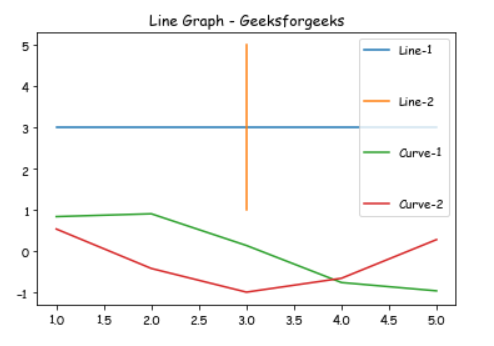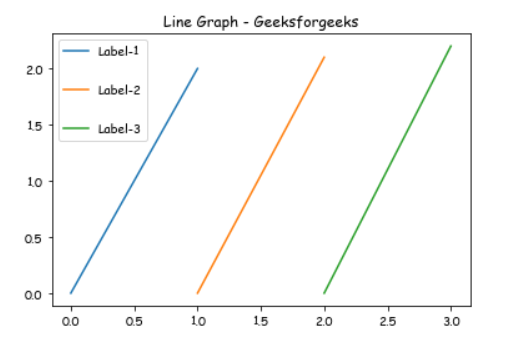# How Change the vertical spacing between legend entries in Matplotlib?

Prerequisites: Matplotlib

In this article, we will see how can we can change vertical space between labels of a legend in our graph using matplotlib, Here we will take two different examples to showcase our graph.

Approach:

• Import required module.
• Create data.
• Change the vertical spacing between labels.
• Normally plot the data.
• Display plot.

Implementation:

Example 1:

In this example, we will draw different lines with the help of matplotlib and Use the labelspacing argument to plt.legend() to change the vertical space between labels.

## Python3

 `# importing package ` `import` `matplotlib.pyplot as plt ` `import` `numpy as np ` ` `  `# create data ` `X ``=` `[``1``, ``2``, ``3``, ``4``, ``5``] ` `Y ``=` `[``3``, ``3``, ``3``, ``3``, ``3``] ` ` `  `# plot lines ` `plt.plot(X, Y, label ``=` `"Line-1"``) ` `plt.plot(Y, X, label ``=` `"Line-2"``) ` `plt.plot(X, np.sin(X), label ``=` `"Curve-1"``) ` `plt.plot(X, np.cos(X), label ``=` `"Curve-2"``) ` ` `  `# Change the label spacing here ` `plt.legend(labelspacing ``=` `3``) ` `plt.title(``"Line Graph - Geeksforgeeks"``) ` ` `  `plt.show()`

Output:Example 2:

In this example, we will draw a Vertical line with the help of matplotlib and Use the labelspacing argument to plt.legend() to change the vertical space between labels.

## Python3

 `# importing package ` `import` `matplotlib.pyplot as plt ` ` `  `#Create data and plot lines. ` `plt.plot([``0``, ``1``], [``0``, ``2.0``], label ``=` `'Label-1'``) ` `plt.plot([``1``, ``2``], [``0``, ``2.1``], label ``=` `'Label-2'``) ` `plt.plot([``2``, ``3``], [``0``, ``2.2``], label ``=` `'Label-3'``) ` ` `  `# Change the label spacing here ` `plt.legend(labelspacing ``=` `2``) ` `plt.title(``"Line Graph - Geeksforgeeks"``) ` ` `  `plt.show()`

Output:Whether you're preparing for your first job interview or aiming to upskill in this ever-evolving tech landscape, GeeksforGeeks Courses are your key to success. We provide top-quality content at affordable prices, all geared towards accelerating your growth in a time-bound manner. Join the millions we've already empowered, and we're here to do the same for you. Don't miss out - check it out now!

Previous
Next